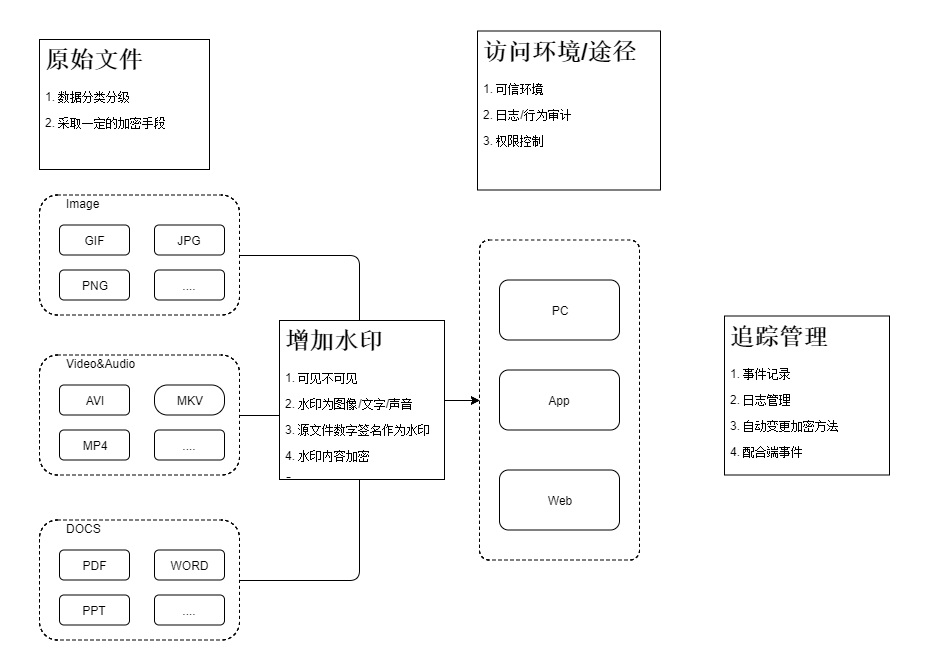# 关于水印这件“小事“

| 分类 安全工程师  | 标签 安全研发  水印

# 已经做的

``````ffmpeg -i input.mp4 -i image.png -filter_complex "[0:v][1:v] overlay=x=10:y=10" -c:a copy output.mp4
``````

``````        with tempfile.TemporaryDirectory() as temp:
# Original uuid generator is not sortable
convert_from_path(self.i_file, output_folder=temp,
thread_count=1, output_file=lambda x: x+1)
for item in sorted(os.listdir(temp)):
self.i_file = os.path.join(temp, item)
it = os.path.join(temp, "{}.png".format(item))

if mark == "text":
self.text(path=it)

if mark == "image":
self.image(path=it)

with open(it, 'rb') as f:
im = Image.open(f)
im.load()
ims.append(im.convert('RGB'))

ims.save(self.o_file, "PDF", resolution=100.0,
save_all=True, append_images=ims[1:])
``````

# 理想中的# 实际上的

• 业务方接入姿势不一，即接入结果不对
• 接入后数据采集姿势不对，即接入结果不对
• 追溯时数据不对，即接入姿势不对
• 追溯时权限和环境难以触达，即接入姿势不对